## Wednesday, March 20, 2013

### The Oblong Square

The Oblong Square
Bro. Doug McCollough

The only way I can express this is to provide the items that I was researching and meditating on when I came into my own personal revelations concerning the relevance of the oblong square, and hope that similar or complementary revelations are unfolded in you.  The ideas presented may be unrelated technically, but they seem to reinforce each other to me. I have found that I need to release the Masonic obsession with the numbers 3, 5, and 7, and adopt an obsession with the numbers 3, 4, and 5.

The Gematria of Moses.

I was studying the Gematria, much of which I personally get from the website Hebrew for Christians .com.  I came across this idea:

“Moses in Hebrew is spelled with the three letters MEM-SHIN-HEH (Mosheh), which has a Gematria of 345 as follows: Mosheh = MEM (40) + SHIN (300) + HEH (5) = 345. On the other hand, the Hebrew name by which God first announces himself to Moses is Eheyeh Asher Eheyeh (“I AM”), with the following Gematria: Eheyeh (21) + Asher (501) + Eheyeh (21) = 543. Thus Moses is a “reflection” of God.  543 to 345.

The 345 Triangle

Two 3, 4, 5 triangles arranged to share a common diagonal.  A “magic square” is shown to indicate where the numbers come from in the two “3, 4, 5” triangles. I leave it to you to get into magic squares if you’re interested.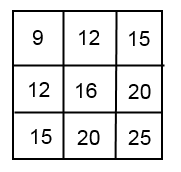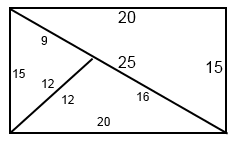My interest in this particular magic square, is the rows (or columns) include numbers that prove out Euclid’s 47th Proposition when applied as a, b, and c. You see the 9, 16, and 25 in the diagonal of the magic square, and know their square roots to be 3, 4, and 5. You know that 3 squared, plus 4 squared = 5 squared.  What I did not think of until seeing it visually is that each 345 triangle can continue to be replicated ever smaller or ever larger in a microcosm/macrocosm relationship “ad infinitum”.Source: http://www.freemasons-freemasonry.com/euclid_proposition.html

The point of the triangles is that when two are placed in mirror positions, they appear to form an oblong square.  This is consistent with the concept of “as above, so below”. So, from that point, whenever I see Euclid’s 47th Proposition shown on a Masonic Jewel or symbol, I now think of an oblong square.

The Divine Proportion

This may not be related to the so-called “Divine Proportion” at all. And I recognize that this proportion does not necessarily align with the Pythagorean Theorem of a squared + b squared = c squared, but follow along with me.  My information on the Divine Proportion comes in part from the excellent paper “Euclid, The Point Within the Circle, The Vesica Pisces, and Divine Proportion” by Bro. William Steve Burkle, KT., 32.

http://www.freemasons-freemasonry.com/divine_proportion.html

The Divine Proportion, The Golden Proportion, The Golden Mean, Phi.

“The Divine Proportion refers to a certain proportional relationship between the length of two lines when that one line is divided into its mean and extreme ratio (i.e. when the ratio of the short part to the long part is the same as the long part to the whole).”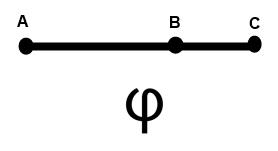Pythagorean Number

I was then studying the excellent book “Mathematical Mysteries” by Calvin Clawson, where I learned the following. According to the Pythagoreans:

There are “Triangular Numbers” - Those that can be laid out (originally as pebbles) as triangles (3, 6, 10, etc).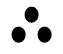There are “Square Numbers” - Those that can be laid out as a square, or more specifically for which adding an “L” shaped “gnomon” forms the next square number.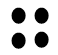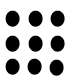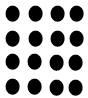And then there are “Oblong Numbers”. “When we add consecutive even numbers beginning with 2, we get numbers that can be formed into a rectangle where the length of the sides differed by one. Such numbers were called Oblong.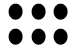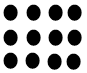Every oblong number is the sum of two triangular numbers (3 over 3, 6 over 6, 10 over 10, etc.).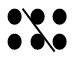Conclusion (and this is a stretch)

There is a relationship between the oblong number, the 345 triangle (exemplified by Euclid’s 47th Proposition), The Divine Proportion (notated by the Greek symbol/letter Phi), and the ideal of eternity (see the relationship of Phi to Fractals) or eternal life.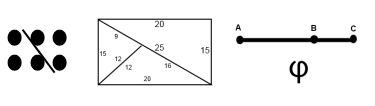My ultimate conclusion is that we uplift the oblong form in order to further focus on the union of opposing forces (two triangular numbers), which exists throughout masonic symbolism, and of course the symbolism of all initiatic and esoteric systems of philosophy.